Скачать презентацию Over Lesson 7 6 Over Lesson 7

1cb73190448735d6914c06de8ed8c557.ppt

• Количество слайдов: 10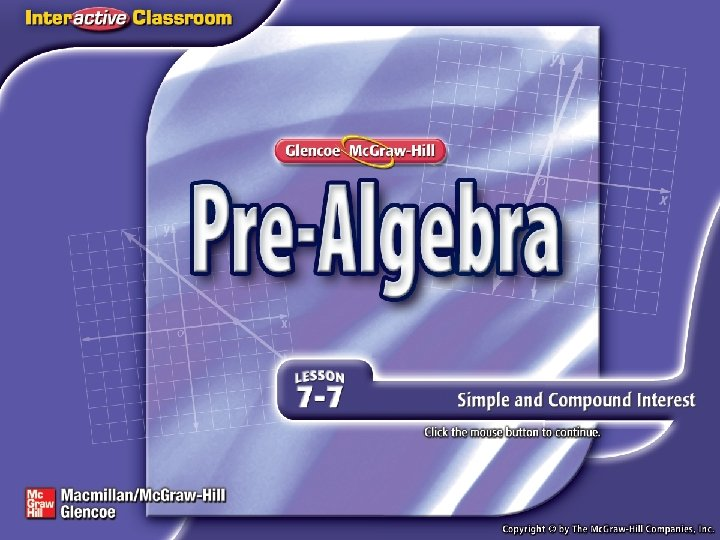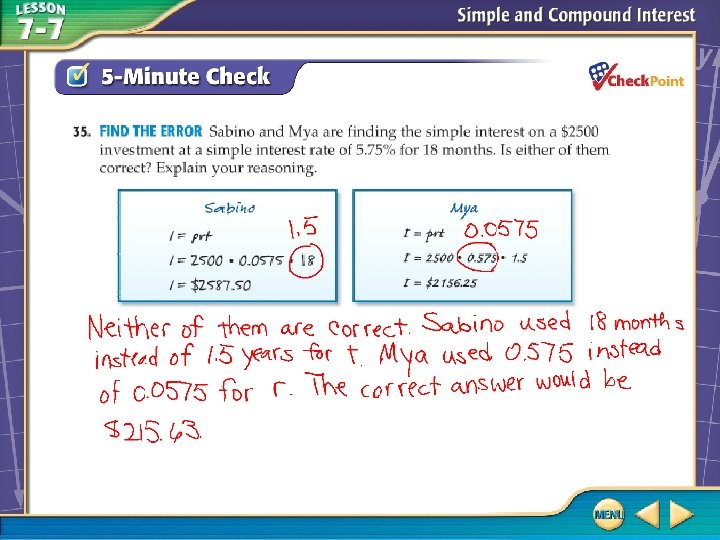Over Lesson 7– 6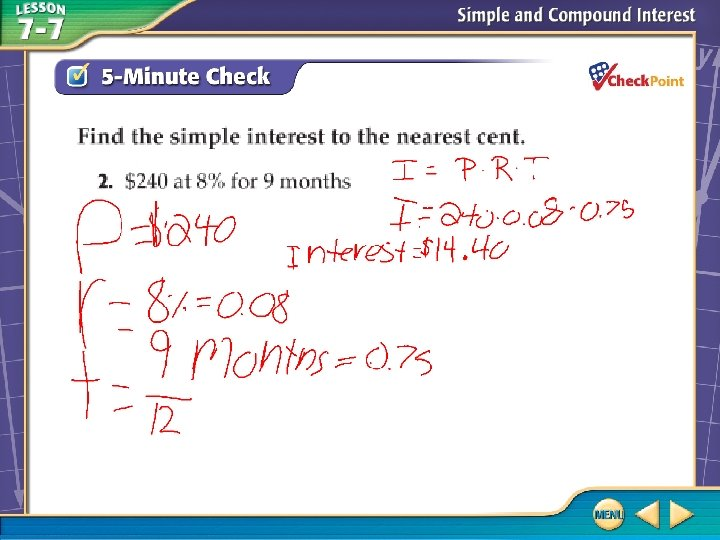Over Lesson 7– 6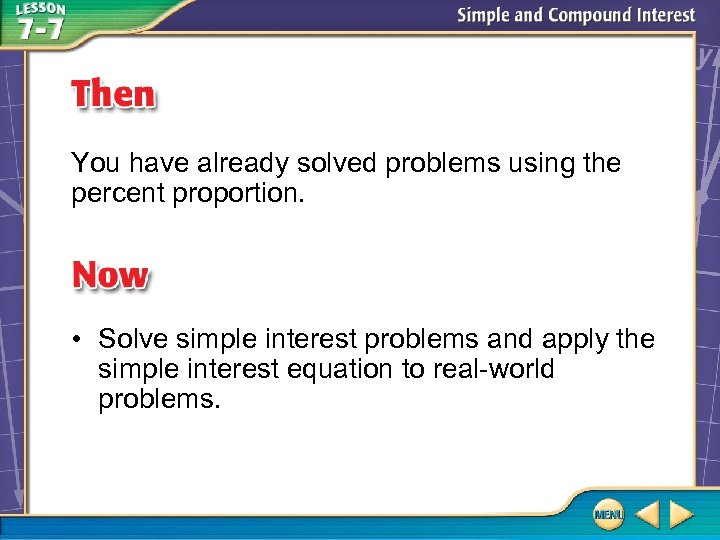You have already solved problems using the percent proportion. • Solve simple interest problems and apply the simple interest equation to real-world problems.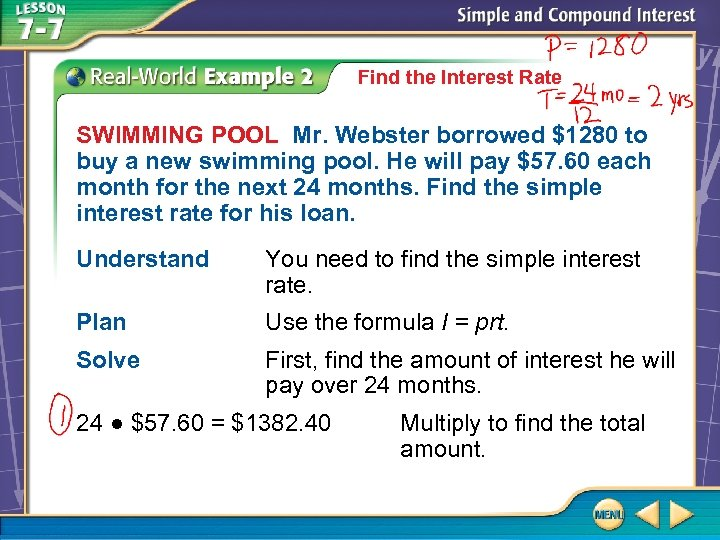Find the Interest Rate SWIMMING POOL Mr. Webster borrowed \$1280 to buy a new swimming pool. He will pay \$57. 60 each month for the next 24 months. Find the simple interest rate for his loan. Understand You need to find the simple interest rate. Plan Use the formula I = prt. Solve First, find the amount of interest he will pay over 24 months. 24 ● \$57. 60 = \$1382. 40 Multiply to find the total amount.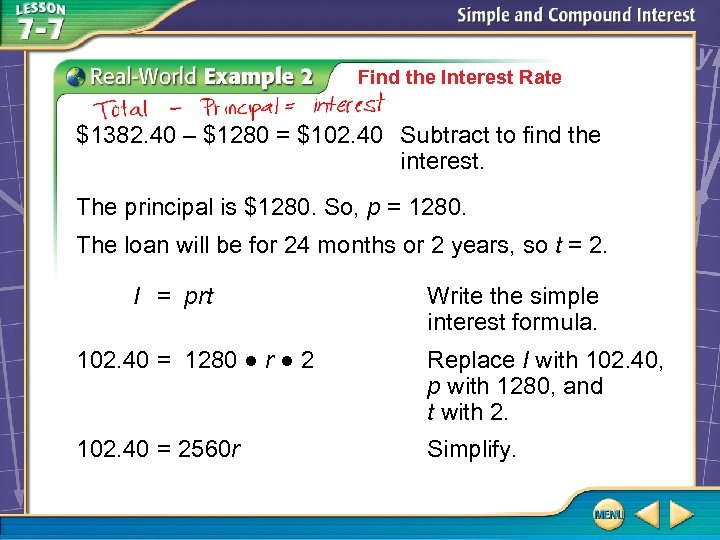Find the Interest Rate \$1382. 40 – \$1280 = \$102. 40 Subtract to find the interest. The principal is \$1280. So, p = 1280. The loan will be for 24 months or 2 years, so t = 2. I = prt Write the simple interest formula. 102. 40 = 1280 ● r ● 2 Replace I with 102. 40, p with 1280, and t with 2. 102. 40 = 2560 r Simplify.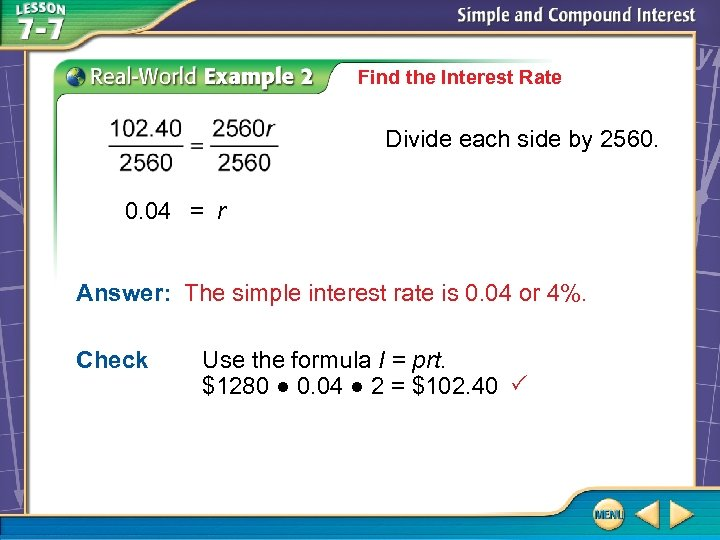Find the Interest Rate Divide each side by 2560. 0. 04 = r Answer: The simple interest rate is 0. 04 or 4%. Check Use the formula I = prt. \$1280 ● 0. 04 ● 2 = \$102. 40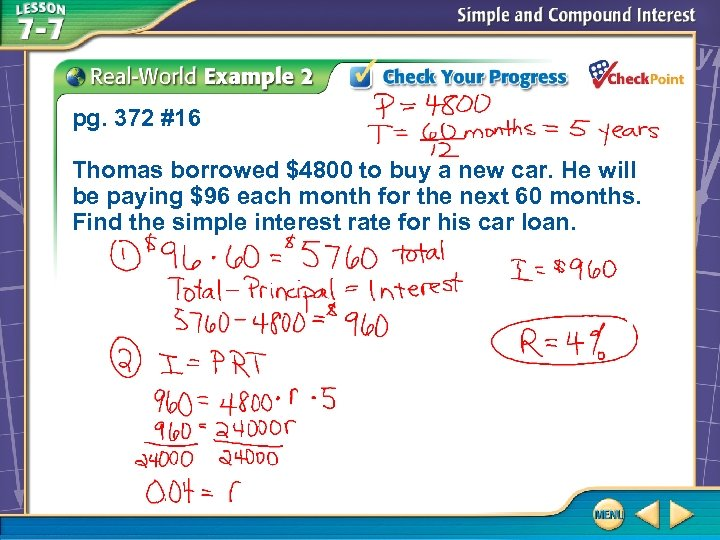pg. 372 #16 Thomas borrowed \$4800 to buy a new car. He will be paying \$96 each month for the next 60 months. Find the simple interest rate for his car loan.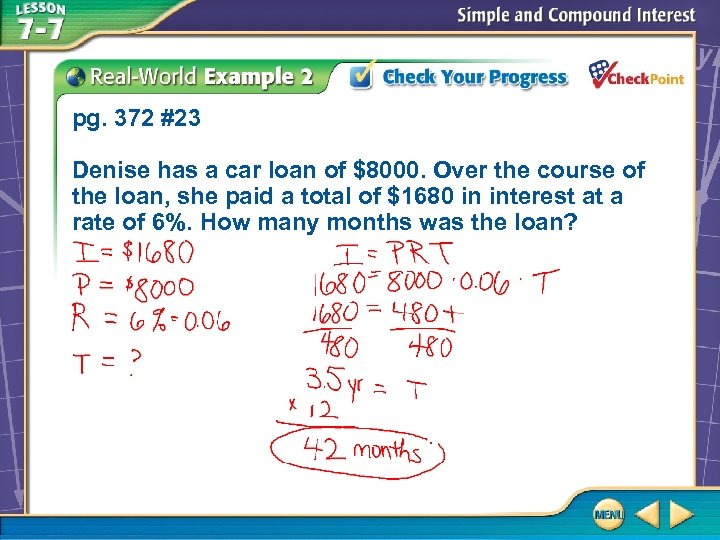pg. 372 #23 Denise has a car loan of \$8000. Over the course of the loan, she paid a total of \$1680 in interest at a rate of 6%. How many months was the loan?pg. 372 #24 A certificate of deposit has an annual simple interest rate of 5. 25%. If \$567 in interest is earned over a 6 year period, how much was invested?